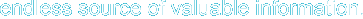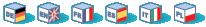# Introduction

These math functions will only handle values within the range of the integer and float types on your computer (this corresponds currently to the C types long resp. double). If you need to handle bigger numbers, take a look at the arbitrary precision math functions.

# Requirements

No external libraries are needed to build this extension.

# Installation

There is no installation needed to use these functions; they are part of the PHP core.

# Runtime Configuration

This extension has no configuration directives defined in php.ini.

# Resource Types

This extension has no resource types defined.

# Predefined Constants

The constants below are always available as part of the PHP core.

Table 1. Math constants

ConstantValueDescription
M_PI3.14159265358979323846Pi
M_E2.7182818284590452354e
M_LOG2E1.4426950408889634074log_2 e
M_LOG10E0.43429448190325182765log_10 e
M_LN20.69314718055994530942log_e 2
M_LN102.30258509299404568402log_e 10
M_PI_21.57079632679489661923pi/2
M_PI_40.78539816339744830962pi/4
M_1_PI0.318309886183790671541/pi
M_2_PI0.636619772367581343082/pi
M_SQRTPI1.77245385090551602729sqrt(pi) [4.0.2]
M_2_SQRTPI1.128379167095512573902/sqrt(pi)
M_SQRT21.41421356237309504880sqrt(2)
M_SQRT31.73205080756887729352sqrt(3) [4.0.2]
M_SQRT1_20.707106781186547524401/sqrt(2)
M_LNPI1.14472988584940017414log_e(pi) [4.0.2]
M_EULER0.57721566490153286061Euler constant [4.0.2]
Only M_PI is available in PHP versions up to and including PHP 4.0.0. All other constants are available starting with PHP 4.0.0. Constants labeled [4.0.2] were added in PHP 4.0.2.

abs -- Absolute value
acos -- Arc cosine
acosh -- Inverse hyperbolic cosine
asin -- Arc sine
asinh -- Inverse hyperbolic sine
atan2 -- Arc tangent of two variables
atan -- Arc tangent
atanh -- Inverse hyperbolic tangent
base_convert -- Convert a number between arbitrary bases
bindec -- Binary to decimal
ceil -- Round fractions up
cos -- Cosine
cosh -- Hyperbolic cosine
decbin -- Decimal to binary
decoct -- Decimal to octal
exp -- Calculates the exponent of e (the Neperian or Natural logarithm base)
expm1 --  Returns exp(number) - 1, computed in a way that is accurate even when the value of number is close to zero
floor -- Round fractions down
fmod -- Returns the floating point remainder (modulo) of the division of the arguments
getrandmax -- Show largest possible random value
hypot --  Calculate the length of the hypotenuse of a right-angle triangle
is_finite -- Finds whether a value is a legal finite number
is_infinite -- Finds whether a value is infinite
is_nan -- Finds whether a value is not a number
lcg_value -- Combined linear congruential generator
log10 -- Base-10 logarithm
log1p --  Returns log(1 + number), computed in a way that is accurate even when the value of number is close to zero
log -- Natural logarithm
max -- Find highest value
min -- Find lowest value
mt_getrandmax -- Show largest possible random value
mt_rand -- Generate a better random value
mt_srand -- Seed the better random number generator
octdec -- Octal to decimal
pi -- Get value of pi
pow -- Exponential expression
rad2deg --  Converts the radian number to the equivalent number in degrees
rand -- Generate a random integer
round -- Rounds a float
sin -- Sine
sinh -- Hyperbolic sine
sqrt -- Square root
srand -- Seed the random number generator
tan -- Tangent
tanh -- Hyperbolic tangent

Hosting by: Hurra Communications Ltd.
Generated: 2007-01-26 18:00:29